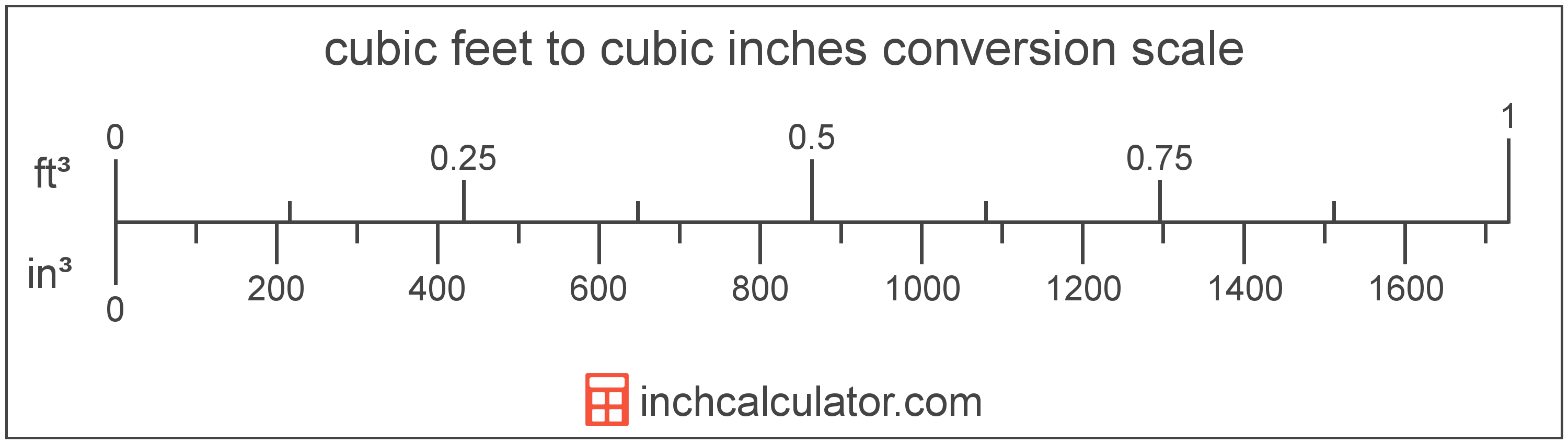# Cubic Inches to Cubic Feet Converter

Enter the volume in cubic inches below to get the value converted to cubic feet.

Results in Cubic Feet:1 in³ = 0.000579 ft³

Do you want to convert cubic feet to cubic inches?

## How to Convert Cubic Inches to Cubic Feet

To convert a measurement in cubic inches to a measurement in cubic feet, divide the volume by the following conversion ratio: 1,728 cubic inches/cubic foot.

Since one cubic foot is equal to 1,728 cubic inches, you can use this simple formula to convert:

cubic feet = cubic inches ÷ 1,728

The volume in cubic feet is equal to the volume in cubic inches divided by 1,728.

For example, here's how to convert 5,000 cubic inches to cubic feet using the formula above.
cubic feet = (5,000 in³ ÷ 1,728) = 2.893519 ft³## What Is a Cubic Inch?

A cubic inch is a unit of volume equal to the space consumed by a cube with sides that are one inch in all directions. One cubic inch is equivalent to about 16.387 cubic centimeters or 0.554 fluid ounces.

The cubic inch is a US customary and imperial unit of volume. A cubic inch is sometimes also referred to as a cubic in. Cubic inches can be abbreviated as in³, and are also sometimes abbreviated as cu inch, cu in, or CI. For example, 1 cubic inch can be written as 1 in³, 1 cu inch, 1 cu in, or 1 CI.

## What Is a Cubic Foot?

The cubic foot is a unit of volume that is equal to the space consumed by a cube having sides one foot on each edge.

The cubic foot is a US customary and imperial unit of volume. A cubic foot is sometimes also referred to as a cubic ft. Cubic feet can be abbreviated as ft³, and are also sometimes abbreviated as cu foot, cu ft, or CF. For example, 1 cubic foot can be written as 1 ft³, 1 cu foot, 1 cu ft, or 1 CF.

You can calculate volume using a cubic footage calculator if you have the dimensions of a space or object.

## Cubic Inch to Cubic Foot Conversion Table

Table showing various cubic inch measurements converted to cubic feet.
Cubic Inches Cubic Feet
1 in³ 0.000579 ft³
2 in³ 0.001157 ft³
3 in³ 0.001736 ft³
4 in³ 0.002315 ft³
5 in³ 0.002894 ft³
6 in³ 0.003472 ft³
7 in³ 0.004051 ft³
8 in³ 0.00463 ft³
9 in³ 0.005208 ft³
10 in³ 0.005787 ft³
20 in³ 0.011574 ft³
30 in³ 0.017361 ft³
40 in³ 0.023148 ft³
50 in³ 0.028935 ft³
60 in³ 0.034722 ft³
70 in³ 0.040509 ft³
80 in³ 0.046296 ft³
90 in³ 0.052083 ft³
100 in³ 0.05787 ft³
200 in³ 0.115741 ft³
300 in³ 0.173611 ft³
400 in³ 0.231481 ft³
500 in³ 0.289352 ft³
600 in³ 0.347222 ft³
700 in³ 0.405093 ft³
800 in³ 0.462963 ft³
900 in³ 0.520833 ft³
1,000 in³ 0.578704 ft³
2,000 in³ 1.1574 ft³
3,000 in³ 1.7361 ft³
4,000 in³ 2.3148 ft³
5,000 in³ 2.8935 ft³
6,000 in³ 3.4722 ft³
7,000 in³ 4.0509 ft³
8,000 in³ 4.6296 ft³
9,000 in³ 5.2083 ft³
10,000 in³ 5.787 ft³

## References

1. National Institute of Standards and Technology, Specifications, Tolerances, and Other Technical Requirements for Weighing and Measuring Devices, Handbook 44 - 2019 Edition, https://nvlpubs.nist.gov/nistpubs/hb/2019/NIST.HB.44-2019.pdf